Normal view

# Applied regression analysis / Norman R. Draper, Harry Smith.

Material type:TextLanguage: English Publisher: New York ; Chichester : John Wiley, c1998Edition: 3rd edDescription: xvii, 706 p. : ill. ; 26 cm. +ISBN: 0471170828 (hbk); 9788126531738; 9780471170822DDC classification: 519.536 LOC classification: QA278.2 | .D7 1998Online resources: WorldCat details | E-book Fulltext
Contents:
Table of contents Preface -- About the software -- Basic prerequisite knowledge -- Fitting a straight line by least squares -- Checking the straight line fit --Fitting straight lines: special topics -- Regression in matrix terms: Straight line case -- The general regression situation -- Extra sums of squares and tests for several parameters being zero -- Serial correlation in the residuals and the Durbin-Watson test -- More on checking fitted models -- Multiple regression: special topics -- Bias in regression estimates, and expected values of mean squares and sums of squares -- On worthwhile regressions, big F's, and R² -- Models containing functions of the predictors, including polynomial models -- Transformation of the response variable -- "Dummy" variables -- Selecting the "Best" regression equation -- Ill-conditioning in regression data -- Ridge regression -- Generalized linear models (GLIM) -- Mixture ingredients as predictor variables -- The geometry of least squares -- More geometry of least squares -- Orthogonal polynomials and summary data -- Multiple regression applied to analysis of variance problems -- An introduction to nonlinear estimation -- Robust regression -- Resampling procedures (Bootstrapping) -- Bibliography -- True/false questions -- Answers to exercises -- Tables -- Index of authors associate with exercises -- Index.
Tags from this library: No tags from this library for this title.Average rating: 0.0 (0 votes)
Item type Current location Collection Call number Copy number Status Date due Barcode Item holdsE-Book
E-book
Non-fiction 519.536 DRA 2011 (Browse shelf) Not For LoanText
Reserve Section
Non-fiction 519.536 DRA 2011 (Browse shelf) C-1 Not For Loan 25684Text
Circulation Section
Non-fiction 519.536 DRA 2011 (Browse shelf) C-2 Checked out 28/08/2019 25686Text
Circulation Section
Non-fiction 519.536 DRA 2011 (Browse shelf) C-3 Available 25685
Total holds: 0
##### Browsing EWU Library shelves, Shelving location: Reserve Section Close shelf browser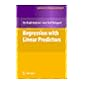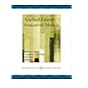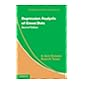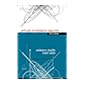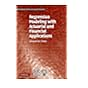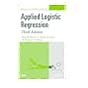519.536 ANR 2010 Regression with linear predictors / 519.536 APP 2005 Applied linear statistical models / 519.536 CAR 2013 Regression analysis of count data / 519.536 DRA 2011 Applied regression analysis / 519.536 FRG 2010 Regression modeling with actuarial and financial applications / 519.536 HOA 2013 Applied logistic regression. 519.536 HOA 2013 Applied logistic regression.

Online version:
Draper, Norman Richard.
Applied regression analysis.
New York : Wiley, c1998
(OCoLC)765771922

Includes bibliographical references (p. 593-603) and indexes.

Table of contents Preface --
About the software --
Basic prerequisite knowledge --
Fitting a straight line by least squares --
Checking the straight line fit --Fitting straight lines: special topics --
Regression in matrix terms: Straight line case --
The general regression situation --
Extra sums of squares and tests for several parameters being zero --
Serial correlation in the residuals and the Durbin-Watson test --
More on checking fitted models --
Multiple regression: special topics --
Bias in regression estimates, and expected values of mean squares and sums of squares --
On worthwhile regressions, big F's, and R² --
Models containing functions of the predictors, including polynomial models --
Transformation of the response variable --
"Dummy" variables --
Selecting the "Best" regression equation --
Ill-conditioning in regression data --
Ridge regression --
Generalized linear models (GLIM) --
Mixture ingredients as predictor variables --
The geometry of least squares --
More geometry of least squares --
Orthogonal polynomials and summary data --
Multiple regression applied to analysis of variance problems --
An introduction to nonlinear estimation --
Robust regression --
Resampling procedures (Bootstrapping) --
Bibliography --
True/false questions --
Answers to exercises --
Tables --
Index of authors associate with exercises --
Index.

Applied Statistics

There are no comments for this item.

to post a comment.
Share

Library Home | Contacts | E-journals
Copyright @ 2011-2019 EWU Library
East West University